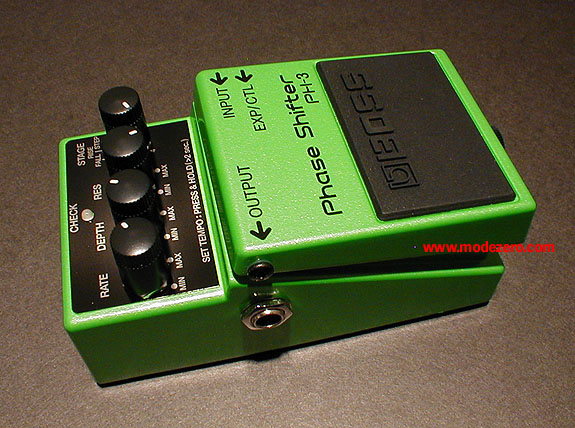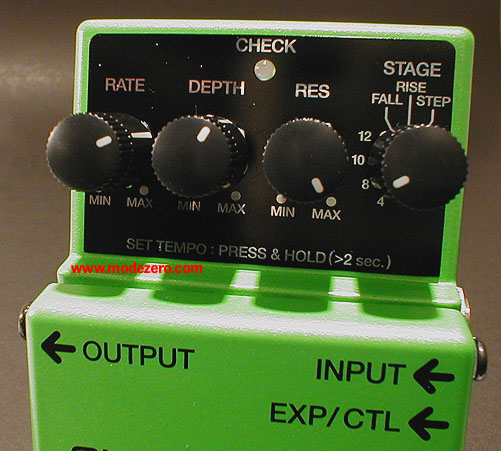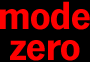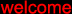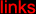(c) 2000-2009

mode zero

boss ph-3 phase shifter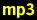guitar, no effectguitar, rate = 1/3, depth = max, res = max, stage = 4guitar, rate = 1/3, depth = max, res = max, stage = 8guitar, rate = 1/3, depth = max, res = max, stage = 10guitar, rate = 1/3, depth = max, res = max, stage = 12guitar, rate = 1/2, depth = 3/4, res = min, stage = 12guitar, rate = 1/2, depth = max, res = max, fallguitar, rate = 1/2, depth = max, res = max, riseguitar, rate = 1/2, depth = max, res = max, stepdrum loop, no effectdrum loop, rate = 1/3, depth = max, res = max, stage = 10drum loop, rate = 1/3, depth = max, res = max, falldrum loop, rate = 1/3, depth = max, res = max, risedrum loop, rate = 1/3, depth = max, res = min, risedrum loop, rate = 3/4, depth = max, res = max, risematrix-1000 pad, no effectmatrix-1000 pad, rate = 1/4, depth = max, res = max, stage = 4matrix-1000 pad, rate = 1/4, depth = max, res = max, stage = 8matrix-1000 pad, rate = 1/4, depth = 3/4, res = min, stage = 8matrix-1000 pad, rate = 1/4, depth = max, res = max, stage = 10matrix-1000 pad, rate = 1/4, depth = max, res = max, stage = 12matrix-1000 pad, rate = 1/4, depth = 3/4, res = min, stage = 12matrix-1000 pad, rate = 1/4, depth = max, res = max, fallmatrix-1000 pad, rate = 1/4, depth = max, res = min, fallmatrix-1000 pad, rate = 1/4, depth = max, res = max, risematrix-1000 pad, rate = 1/4, depth = max, res = min, risematrix-1000 pad, rate = 2/3, depth = 1/2, res = max, risematrix-1000 pad, rate = 2/3, depth = 1/2, res = min, risematrix-1000 pad, rate = 1/2, depth = max, res = max, stepmatrix-1000 pad, rate = 1/3, depth = 2/3, res = max, stepmatrix-1000 pad, rate = 3/4, depth = 1/2, res = 1/2, stage = 8klb loop, no effectklb loop, rate = slow, depth = max, res = max, stage = 10klb loop, rate = slow, depth = max, res = max, riseklb loop, rate = slow, depth = max, res = max, fall